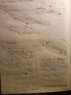# Resistive force against a bullet

Deckname
Homework Statement:
A bullet of mass 20g hits a steel plate at 600m/s and ricochets in the direction of CD at 500m/s. The bullet makes an indentation in the plate of length 50mm with an entering and exiting angle of beta. The angle alpha between the leaving velocity vector and the horizon is 30°

Calculate the average force of resistance the steel plate is providing the bullet with.

Solution: 9.46x10^7 N
Relevant Equations:
(1/2)mv^2
I tried getting to the solution by the principle of conservation of energy. What goes in, must go out. If the final velocity, ie. the final kinetic energy is lower than what we started with, that energy difference must've been used to overcome the friction that lasted over some distance L, meaning that the energy spent to overcome the friction is equal to the work done by the friction force F over the distance L. My way of thinking is now that KE(i)-KE(f)=F*L. It turns out I'm wrong and I don't really know how else I can attempt to arrive at the correct solution, which is magnitudes above what I keep getting.

#### Attachments

•20190411_232610.jpg
78.6 KB · Views: 192

Homework Helper
Gold Member
2022 Award
I tried getting to the solution by the principle of conservation of energy. What goes in, must go out. If the final velocity, ie. the final kinetic energy is lower than what we started with, that energy difference must've been used to overcome the friction that lasted over some distance L, meaning that the energy spent to overcome the friction is equal to the work done by the friction force F over the distance L. My way of thinking is now that KE(i)-KE(f)=F*L. It turns out I'm wrong and I don't really know how else I can attempt to arrive at the correct solution, which is magnitudes above what I keep getting.
"Force of resistance" may be ambiguous. The plate resists being penetrated, so it might mean the average force exerted.

Imagine the bullet had entered a smoothly curving tube, deflecting the bullet with no loss of speed. Would you conclude the tube had exerted no force on the bullet?

(But I get a smaller answer too.)

Edit: are you sure the bullet is initially moving horizontally?

Last edited:
Deckname
Hey, thanks for the reply. Appreciate it.

I translated this problem from my native language, but yeah I'm not too sure either, but literally translated it means calculate the middle force of resistance, which I suppose means the average force of resistance, if we assume the force of friction getting lower the less momentum the bullet has, if that's how it works. I have to admit, I don't have a strong background in physics and have only started learning it a year ago, depending on what my course requires me.

I tried a different approach though, I treated the force of friction and the change in momentum as an impulse problem, calculated the time it took the bullet to end up from one side of the plate to the other, ie I calculated the contact time. And arrived at pretty much the same result I have already posted in my picture.

Regarding the entering angle of the velocity vector, it doesn't explicitly state that it's horizontal, but I can clearly see it in the picture I drew it from.

Also, there's a fair possibility that the solution provided by my teacher is wrong, since this is the case with a few other problems I've been assigned as well. But then again, usually the answers deviate by a few hundreds of Newtons sometimes, and not by a few orders of a magnitude like here, so that's what's getting me worked up.

I can't seem to get nowhere near his answer, assuming it's correct.